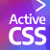There are no tracking or monitoring cookies on this site. There are only cookies used for documentation examples related to cookies.
Processing...
This may take a few seconds.

# @for

Syntax

`(event selector:event) {    (...)    @for (control variable) from ([-]starting number) to ([-]ending number)[ step ([-]step number)] {        (...)        (target selector) {            (...)            @for (control variable) from ([-]starting number) to ([-]ending number)[ step ([-]step number)] {                (action commands)            }            (...)        }        (...)    }    (...)}`

The command used for basic looping with numbers is the @for statement. @for is not exactly equivalent to the for loop in JavaScript, although it is similar.

The variable that stores the @set value during iteration (the control variable) is automatically set and scoped per the scoping rules.

Loops in Active CSS can be placed around either target selectors or action commands.

control variable = This is the variable that contains the current number in the loop. This does not have curly brackets. This variable does not need to be declared before its appearance in the loop.

[-]starting number = This is where the loop starts. This can be a positive number (eg. 3), a negative number (eg. -3), a number with up to 5 decimal places (eg. 1.00001), a variable that contains a number, or a JavaScript expression. When using a variable here, it should have curly brackets.

[-]ending number = This is where the loop ends, and the final iteration of the loop will include this number. This has the same syntax rules as the starting number.

[-]step number [optional] = The incremental or decremental value to apply to the control variable as the loop progresses. This has the same syntax rules as the starting number.

If the parameter step is not mentioned, the step number default is positive 1.

When moving from a positive starting number to a negative ending number, the step number should be negative (eg. -1).

See this example in Active CSS (which would be placed inside an event declaration):``@for \$n from 1 to 10 {    console-log: "value is {\$n}";}/* Outputs the digits 1 to 10 into the console. */``

That is the same as doing this in JavaScript:``for (let n = 1; n <= 10; n++) {    console.log('value is ' + n);}// Outputs the digits 1 to 10 into the console.``

In Active CSS you can also use variables to do the same thing:``\$start: 1;\$end: 10;@for \$n from \$start to \$end {    console-log: "value is {\$n}";}/* Outputs the digits 1 to 10 into the console. */``

If you want to iterate an array in a similar way to JavaScript, you can use the @for statement like the following example (or you could just use the @each statement, which is easier to read):``\$myArr: [10, 20, 30, 40, 50];@for n from 0 to \$myArr.length - 1 {    console-log: "item is: {\$myArr[n]}";}``

Another example, this time progressing from 10 to 1:``@for \$n from 10 to 1 step -1 {    console-log: "value is {\$n}";}/* Outputs into the console the digits from 10 to 1, including the final 1. */``

Nested @for loops are allowed (see the example at the bottom of this page).

@for loops and their inner commands, like all the commands in Active CSS, run in sequence, flowing downwards in the config, and then start again from the top until the looping has completed.

Very large number of iterations may produce unpredictable results. A very large number of iterations, above several thousand, may crash the browser on a slower device. This is because of the processing power involved with reading and running action commands. Active CSS runs fast, but it does not run at a low enough level to handle thousands of iterations.

There now follows examples of possible uses of the @for statement, including use of the break, continue, exit and exit-target control flow commands.

### @for - continue

continue puts the control flow immediately back to the top of the loop.

### @for - break

break puts the control flow immediately after the loop (it breaks out of the loop).

### @for - exit

exit breaks out of the whole event. Nothing else in the event will run.

### @for - exit-target

exit-target breaks out of all actions inside the target selector.